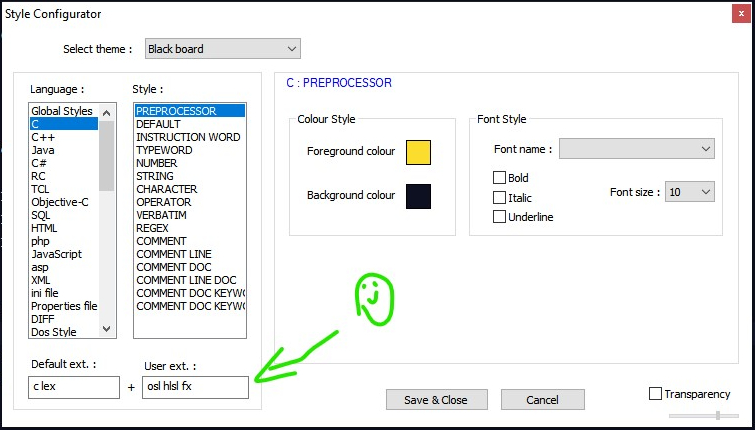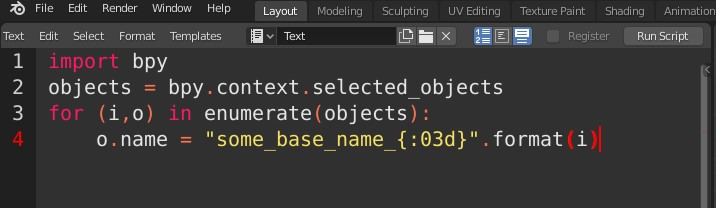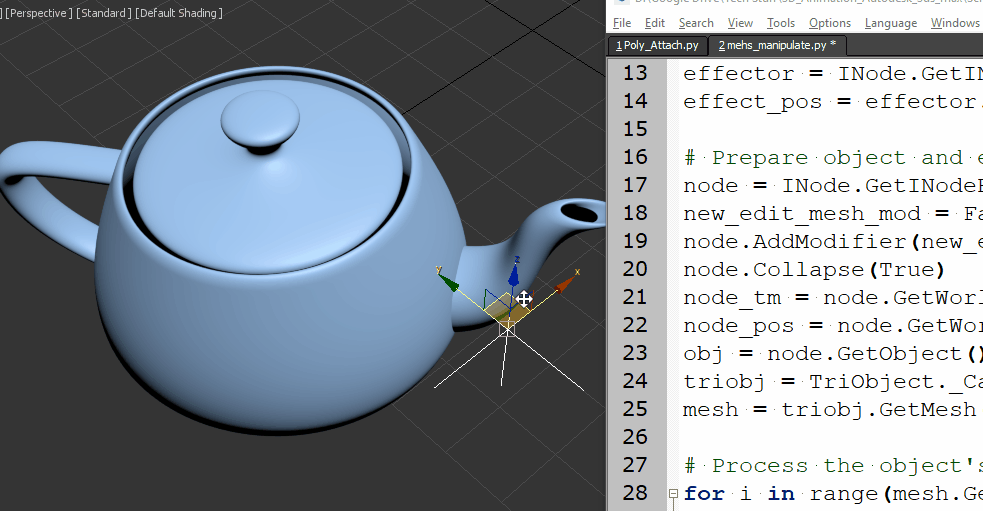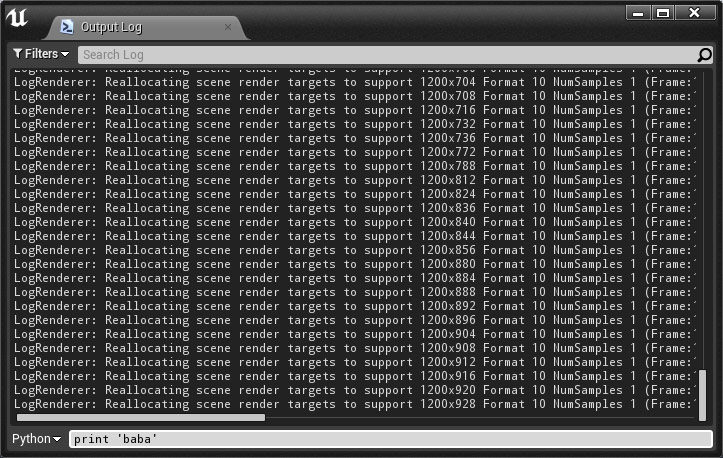## A collection of Python snippets for 3D

If you’re interested in taking the first step into Python for 3D software,
Or simply would like to browse some script examples, your welcome to visit my Gist page,
It contains a useful library of Python code example for Blender, Maya, 3ds max and Unreal engine:
https://gist.github.com/CGLion

## Blender Python – Reading mesh UV data

Software:
Blender 2.83

Simple example code for reading mesh UV data.

Link to this code snippet on gist

Note that there are typically many more mesh loops than vertices.
*Unless the case is a primitive undivided plane..

``````import bpy
access mesh data:
obj = bpy.context.active_object
mesh_data = obj.data
mesh_loops = mesh_data.loops
uv_index = 0
iterate teh mesh loops:
for lp in mesh_loops:
# access uv loop:
uv_loop = mesh_data.uv_layers[uv_index].data[lp.index]
uv_coords = uv_loop.uv
print('vert: {}, U: {}, V: {}'.format(lp.vertex_index,      uv_coords, uv_coords))``````

## Associate file extensions to language in Notepad++

Software:
Notepad++ 7.8.6

If your writing code in languages that are based on a common language but their files names have an uncommon extension, for example, the 3D shading languages OSL, and HLSL, which are written in C syntax but have .osl and .hlsl, or .fx as file name extensions,
Notepad++ wont automatically recognize that the code in these files is actually C language and present their syntax properly.

To set Notepad++ to recognize specific file associations with a wanted language:

1. Goto:
Settings > Style Configurator:2. In the Style Configurator window:
Select the wanted language in the language list on the left,
And in the User ext. field, type a list of file extensions separated by spaces:
These file extensions will be interpreted as the selected language.## Python for 3ds max – Loading an image file and reading pixel values

Software:
3ds max 2020

An example of loading and displaying an image file using Python for 3ds max:
* The EXR image file is located in in the same directory with the 3ds max file in this case.
```from MaxPlus import BitmapManager
image_file_path = r'BG_park_A.exr'
bmp_storage = MaxPlus.Factory.CreateStorage(17)
bmp_info = bmp_storage.GetBitmapInfo()
bmp_info.SetName(image_file_path)
bmp = BitmapManager.Load(bmp_info)
bmp.Display()```

Script explanation line by line:
1. Import the BitmapManager class needed to load image files.
2. Set a variable containing the path to the image file
3. Call the MaxPlus.Factory class’s CreateStorage method to initiate a BitmapStorage object.
This is embarrassing IMO..
And it may very well be that I simply didn’t find the correct way it should be done..
I couldn’t find any other way to independently initiate the BitmapInfo object needed for loading the image, other than Initiating a BitmapStorage object and getting referece to its BitmapInfo object. (the BitmapInfo class has no constructor..)
* If you know a better method to do this I’ll be very grateful if you take the time to comment.
Note:
that the 17 integer argument we supply sets the storage to be compatible with:
32-bit floating-point color depth format (without an alpha channel).
See list of other color format options in this example here:
https://help.autodesk.com/view/3DSMAX/2020/ENU/?guid=__developer_using_maxplus_creating_a_bitmap_html
* They wrote a class containing convenient named constants of the integer arguments (see example code below).
* In this example of creating the BitmapStorage just as a way to generate a BitmapInfo object the actual format you’l supply doesn’t matter, but you can’t use a format that can’t be written to like 8 for example (see list below)
4. Get a reference to the BitmapInfo object contained in the BitmapStorage object.
5. Setting the name property (full file path) of the BitmapInfo object.
6. Loading the image.
7. Displaying the image in 3ds max‘s image viewer window.

Example code for a BitmapStorage format constants container class:

* This example’s source is in the 3ds max help Python examples:
https://help.autodesk.com/view/3DSMAX/2020/ENU/?guid=__developer_using_maxplus_creating_a_bitmap_html
```class BitmapTypes(object):
BMM_NO_TYPE = 0 # Not allocated yet
BMM_LINE_ART = 1 # 1-bit monochrome image
BMM_PALETTED = 2 # 8-bit paletted image. Each pixel value is an index into the color table.
BMM_GRAY_8 = 3 # 8-bit grayscale bitmap.
BMM_GRAY_16 = 4 # 16-bit grayscale bitmap.
BMM_TRUE_16 = 5 # 16-bit true color image.
BMM_TRUE_32 = 6 # 32-bit color: 8 bits each for Red, Green, Blue, and Alpha.
BMM_TRUE_64 = 7 # 64-bit color: 16 bits each for Red, Green, Blue, and Alpha.
BMM_TRUE_24 = 8 # 24-bit color: 8 bits each for Red, Green, and Blue. Cannot be written to.
BMM_TRUE_48 = 9 # 48-bit color: 16 bits each for Red, Green, and Blue. Cannot be written to.
BMM_YUV_422 = 10 # This is the YUV format - CCIR 601. Cannot be written to.
BMM_BMP_4 = 11 # Windows BMP 16-bit color bitmap. Cannot be written to.
BMM_PAD_24 = 12 # Padded 24-bit (in a 32 bit register). Cannot be written to.
BMM_LOGLUV_32 = 13 BMM_LOGLUV_24 = 14
BMM_LOGLUV_24A = 15 BMM_REALPIX_32 = 16 # The 'Real Pixel' format.
BMM_FLOAT_RGBA_32 = 17 # 32-bit floating-point per component (non-compressed),
RGB with or without alpha
BMM_FLOAT_GRAY_32 = 18 # 32-bit floating-point (non-compressed), monochrome/grayscale
BMM_FLOAT_RGB_32 = 19
BMM_FLOAT_A_32 = 20```

Reading pixel values from the image:
```bmp_storage = bmp.GetStorage()
hdr_pixel = bmp_storage.GetHDRPixel(3000,200)
print(hdr_pixel)```
1. Get reference to the Bitmap‘s BitmapStorage object.
* in this case, writing over the BitmapStorage object we created earlier just to get the BitmapInfo object..
2. Read the pixel value.

Note:

When copying and pasting a script from this example, the indentation may not be pasted correctly.

Related:

## Python for Blender – Batch renaming objects

Software:
Blender 2.8 | Python 3.7

Some useful short Python snippets for batch re-naming objects in Blender:

1. Remove last 4 characters from selected object names:
```import bpy
objects = bpy.context.selected_objects
for o in objects:
o.name = o.name[:-4]```2. Rename all selected objects to a set base name followed by a 3 digit numeric suffix:
```import bpy
objects = bpy.context.selected_objects
for (i,o) in enumerate(objects):
o.name = "some_base_name_{:03d}".format(i)```3. Prefix all selected objects name with their Blender data type like renaming “SomeModel” to “MESH_SomeModel” or for example:
```import bpy
objects = bpy.context.selected_objects
for (i,o) in enumerate(objects):
o.name = "{}_{}".format(o.type,o.name)```* note that when copying and pasting a script from this example, the indentation may not be pasted correctly.

Note:
Blender 2.8 has a robust batch renaming utility that is invoked by pressing Ctrl + F2 so we don’t have to write scripts to do batch renaming of objects:## Python for 3ds max – Select objects of type

Software:
3ds max 2020

Continuing this example,
If you need to select objects (nodes) of a certain type i.e. lights, cameras etc.
You can use the INode class’s GetObject() method to get a reference to the node’s object, or “modified object” in 3ds max terms, and use the object’s GetSuperClassID() method to get the integer id representing the object’s superclass.

In addition, the MaxPlus SuperClassIds class contains convenient constants that can be used to avoid having to check and remember the superclasses numeric ids.
See reference here:
https://help.autodesk.com/view/3DSMAX/2017/ENU/?guid=__py_ref_class_max_plus_1_1_super_class_ids_html

An example of a script that selects all light objects in the scene:

```from MaxPlus import SuperClassIds
from MaxPlus import SelectionManager

def scene_objects():
def list_children(node):
list = []
for c in node.Children:
list.append(c)
list = list + list_children(c)
return list
return list_children(MaxPlus.Core.GetRootNode())

for o in scene_objects():
obj = o.GetObject()
if obj.GetSuperClassID() == SuperClassIds.Light:
SelectionManager.SelectNode(o, False)```

* note that when copying and pasting a script from this example, the indentation may not be pasted correctly.

## Python for 3ds max – Mesh manipulation

Software:
3ds max 2019

An example of creating a mesh ripple deformation using Python for 3ds max:Script steps:

1. Define the effect intensity and a helper point object that will serve as the effect center.
2. Collapse the object to an Editable-Mesh so its vertices will be accessible by Python.
Note that the Node‘s Object has to be cast as a TriObject, to access the object’s Mesh data.
3.  Loop through the Mesh’s vertices, get their world position, and set a new Z position as a sine function of the distance from the effect center.
```import math
from MaxPlus import Factory
from MaxPlus import ClassIds
from MaxPlus import INode
from MaxPlus import TriObject
from MaxPlus import Matrix3
from MaxPlus import Point3

# Intensity:
effecr_mult = 1.0

# Effect center:
effector = INode.GetINodeByName('Point001')
effect_pos = effector.GetWorldPosition()

# Prepare object and eccess it's mesh data:
node = INode.GetINodeByName('Teapot001')
new_edit_mesh_mod = Factory.CreateObjectModifier(ClassIds.Edit_Mesh)
node.AddModifier(new_edit_mesh_mod)
node.Collapse(True)
node_tm = node.GetWorldTM()
node_pos = node.GetWorldPosition()
obj = node.GetObject()
triobj = TriObject._CastFrom(obj)
mesh = triobj.GetMesh()

# Process the object's vertices:
for i in range(mesh.GetNumVertices()):
# Get vertex in world space
vert_pos = mesh.GetVertex(i)
vert_world_pos = node_tm.VectorTransform(vert_pos)
vert_world_pos = vert_world_pos + node_pos
# Get vertex distance from effect center:
diff_vec = vert_world_pos - effect_pos
diff_vec.Z = 0
dist = diff_vec.GetLength()
# Set new vertex position:
mesh.SetVert(i,vert_pos.X,vert_pos.Y,vert_pos.Z + math.sin(dist)*effecr_mult)```

* note that when copying and pasting a script from this example, the indentation may not be pasted correctly.

Related Post:
Python for 3ds max – Animated Mesh

## UE4 – Python – Importing assets

Software:
Unreal Engine 4.20Importing assets into a project is done using the import_asset_tasks() function which is a member of the unreal.AssetTools class.
A reference to the AssetTools class is created by calling the get_asset_tools() function which is a member of the unreal.AssetToolHelpers class.
The import_asset_tasks() function requires a list of unreal.AssetImportTask objects as an argument, each unreal.AssetImportTask object in the supplied list represents the import action of a single asset, and contains properties needed for the import operation.
Asset import properties are set using the set_editor_property() function which is called through the AssetImportTask object.
Available asset import properties are listed here:
https://api.unrealengine.com/INT/PythonAPI/class/AssetImportTask.html

In the following example a specified texture file is imported into the project and stored in the Content(Game) > Textures folder.
* If the folder doesn’t exist it will be created.

```import unreal
AssetTools = unreal.AssetToolsHelpers.get_asset_tools()
AssetImportTask = unreal.AssetImportTask()
AssetImportTask.set_editor_property('filename', "D:\Wood_Red_A.jpg")
AssetImportTask.set_editor_property('destination_path', '/Game/Textures')
AssetTools.import_asset_tasks([AssetImportTask])```

The following example imports all the JPG files from folder: D:\ into the project, stores the new assets in Content(Game)\Textures folder and saves them:

```from os import listdir
from os.path import isfile, join
import unreal
dir = "D:\\"
files = [f for f in listdir(dir) if isfile(join(dir, f)) and f[-3:]=='jpg']
AssetTools = unreal.AssetToolsHelpers.get_asset_tools()

import_tasks = []
for f in files:
print join(dir, f)
AssetImportTask = unreal.AssetImportTask()
AssetImportTask.set_editor_property('filename', join(dir, f))
AssetImportTask.set_editor_property('destination_path', '/Game/Textures')
AssetImportTask.set_editor_property('save', True)
import_tasks.append(AssetImportTask)

AssetTools.import_asset_tasks(import_tasks)```

* note that when copying and pasting a script from this example, the indentation may not be pasted correctly.

Related:

## UE4 – Python Scripting – how to start

Software:
Unreal Engine 4.20

1. Go to:
Edit > Plugins > Scripting
And enable the Python Editor Script Plugin.
* also recommended to enable Editor Scripting Utilities,
And Sequencer Scripting plugins2. Restart the UE4 Editor.
3. Open:
Window > Developer Tools > Output Log4. Switch the command-line mode from Cmd to Python, write Python commands and press Enter to execute them:5. Or in Cmd mode, write ‘py‘ with a path to a Python script file, and hit Enter to execute the script:Links:

1. Scripting the Editor using Python:
https://docs.unrealengine.com/en-us/Editor/ScriptingAndAutomation/Python
2. UE4 Python API reference:
https://api.unrealengine.com/INT/PythonAPI/

Examples:

## Python for 3ds max – Animated Mesh

Software:
3ds max 2019

This is an example of procedurally animating a mesh’s vertices via Python script.Notes:
1. The model has to be converted to Editable Mesh before the script is run.
* unless the scrip will be extended to do it.
2. The model must be selected for the script to work.

```import MaxPlus
import math
from MaxPlus import INode
from MaxPlus import TriObject
from MaxPlus import SelectionManager
from MaxPlus import Factory
from MaxPlus import Animation
from MaxPlus import Point3
from MaxPlus import Control

ticks_frame = 160

#Selection
sel = []
for n in SelectionManager.Nodes:
sel.append(n)
node = sel

#Setup Controllers
obj = node.GetObject()
Tri = TriObject._CastFrom(obj)
mesh = Tri.GetMesh()
num_verts = mesh.GetNumVertices()
mesh_anim = obj.GetSubAnim(0)
pnt_ctrl = Factory.CreateDefaultMasterPointController()
node.AssignController(pnt_ctrl,1)
for i in range(num_verts):
bezp3 = Factory.CreateDefaultPoint3Controller()
bezp3.SetPoint3Value(mesh.GetVertex(i))
mesh_anim.AssignController(bezp3,i)

#Animation
Animation.SetAnimateButtonState(True)
for t in range(100):
time = t * ticks_frame
Animation.SetTime(time)
mesh_anim.AddNewKey(time,0)
for i in range(num_verts):
vert_anim = mesh_anim.GetSubAnim(i)
vert_ctrl = Control._CastFrom(vert_anim)
vert_val = mesh.GetVertex(i)
vert_val.SetZ(vert_val.GetZ() + math.sin(((Animation.GetTime()*0.5)/(ticks_frame))+i))
vert_ctrl.SetPoint3Value(vert_val)
Animation.SetAnimateButtonState(False)```

* note that when copying and pasting a script from this example, the indentation may not be pasted correctly.